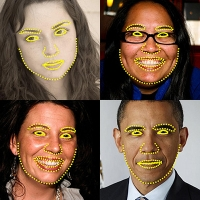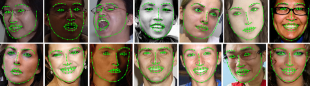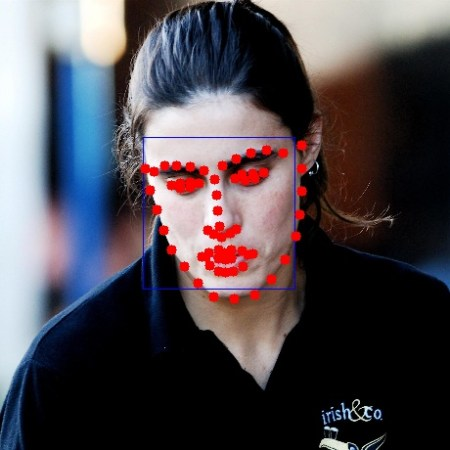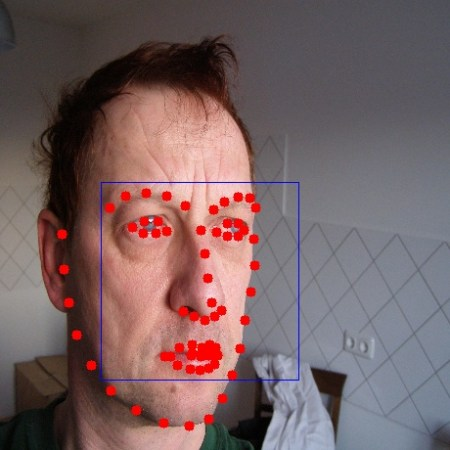OpenCV  3.4.0 Open Source Computer Vision
Face landmark detection in an imageThis application lets you detect landmarks of detected faces in an image. You can detect landmarks of all the faces found in an image and use them further in various applications like face swapping, face averaging etc. This functionality is now available in OpenCV.

// Command to be typed for running the sample

### Description of command parameters {tutorial_face_training_parameters}

• model_filename f : (REQUIRED) A path to binary file storing the trained model which is to be loaded [example - /data/file.dat]
• image i : (REQUIRED) A path to image in which face landmarks have to be detected.[example - /data/image.jpg]
• face_cascade c : (REQUIRED) A path to the face cascade xml file which you want to use as a face detector.

## Understanding codeThis tutorial will explain the sample code for face landmark detection. Jumping directly to the code :

bool myDetector( InputArray image, OutputArray ROIs );
bool myDetector( InputArray image, OutputArray ROIs ){
Mat gray;
std::vector<Rect> faces;
if(image.channels()>1){
cvtColor(image.getMat(),gray,CV_BGR2GRAY);
}
else{
gray = image.getMat().clone();
}
equalizeHist( gray, gray );
face_cascade.detectMultiScale( gray, faces, 1.1, 3,0, Size(30, 30) );
Mat(faces).copyTo(ROIs);
return true;
}

The facemark API provides the functionality to the user to use their own face detector to be used in training.The above code creartes a sample face detector. The above function would be passed to a function pointer in the facemark API.

FacemarkKazemi::Params params;
params.configfile = configfile_name;
Ptr<Facemark> facemark = FacemarkKazemi::create(params);
facemark->setFaceDetector(myDetector);

The above code creates a pointer of the face landmark detection class. The face detector created above has to be passed as function pointer to the facemark pointer created for detecting faces while landmark detection. The above code also loads the image in which landmarks have to be detected.

vector<Rect> faces;
resize(img,img,Size(460,460),0,0,INTER_LINEAR_EXACT);
facemark->getFaces(img,faces);
vector< vector<Point2f> > shapes;
 c++
The above code loads a trained model for face landmark detection and creates a vector to store the detected faces. It then resizes the image to a smaller size as processing speed is faster with small images. It then creates a vector of vector to store shapes for each face detected.
 c++
if(facemark->fit(img,faces,shapes))
{
for( size_t i = 0; i < faces.size(); i++ )
{
cv::rectangle(img,faces[i],Scalar( 255, 0, 0 ));
}
for(unsigned long i=0;i<faces.size();i++){
for(unsigned long k=0;k<shapes[i].size();k++)
cv::circle(img,shapes[i][k],5,cv::Scalar(0,0,255),FILLED);
}
namedWindow("Detected_shape");
imshow("Detected_shape",img);
waitKey(0);
}

The above code then calls the function fit to get shapes of all detected faces in the image and then draws the rectangles bounding the faces and marks the desired landmarks.

### Detection Results